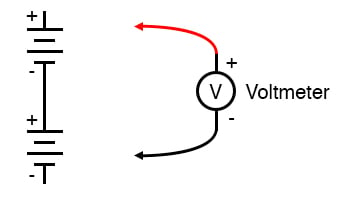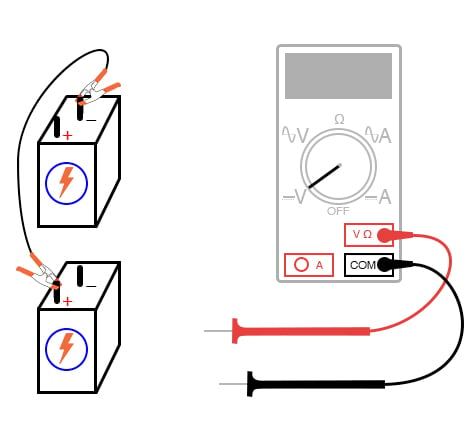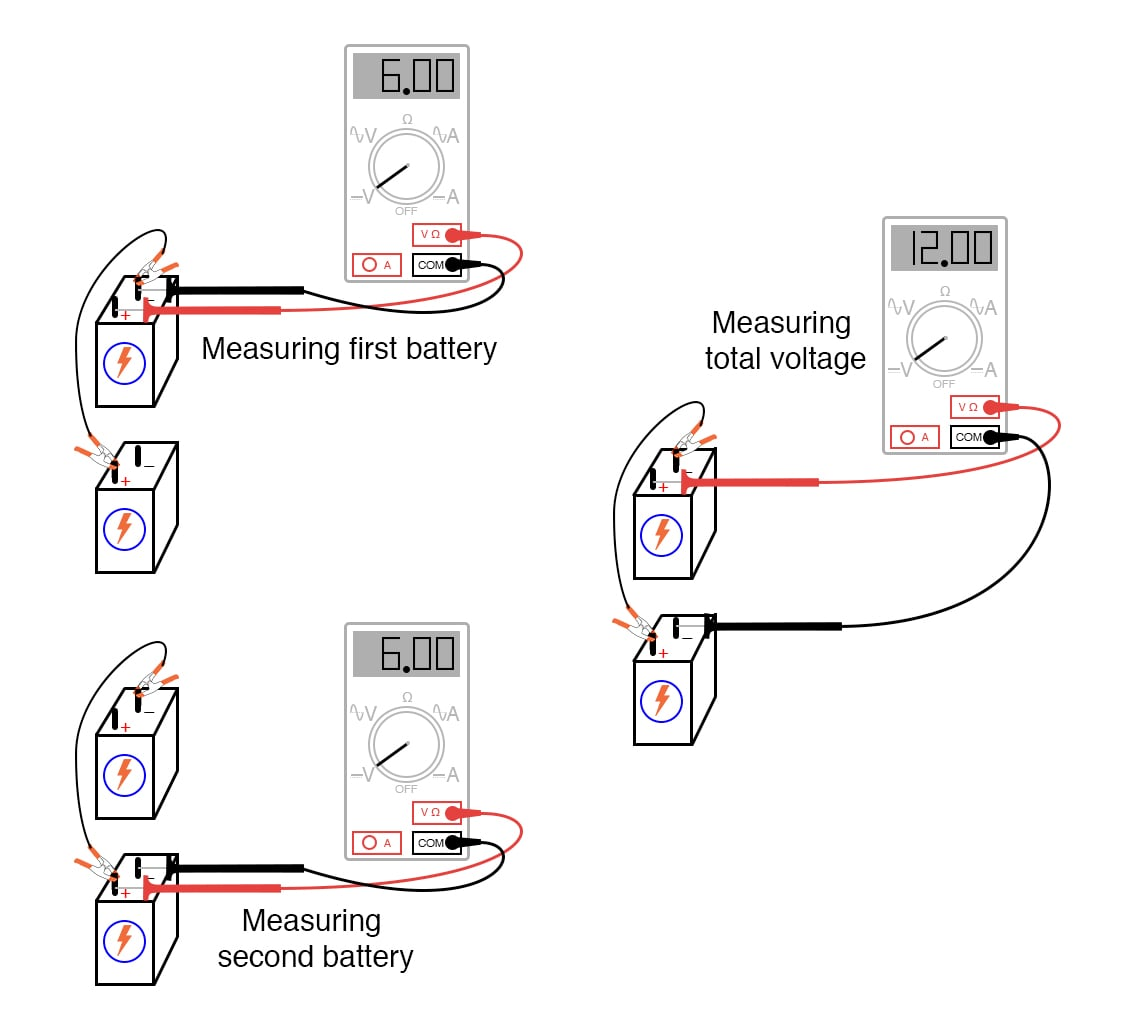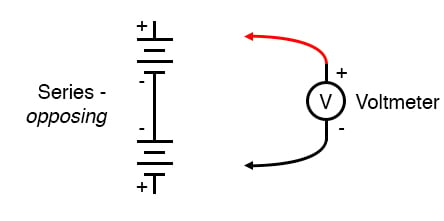Vol. DIY Electronics Projects
Chapter 3 DC Circuit Projects

# DC Lab - Series Batteries

## In this hands-on electronics experiment, you will connect batteries in series and learn the relationship between the individual battery voltages and the total series voltage.

### Project Overview

Connecting batteries in series, as shown in Figure 1, means connecting them in line with each other so that there is but a single path for electrons to flow through them all. If you connect batteries so that the positive terminal of one battery connects to the negative of the other, you will find that their respective voltages add up.### Parts and Materials

• Two 6 V batteries
• One 9 V battery

Note: Any size batteries will work for this experiment, but using batteries of different voltages will make the results more interesting

### Instructions

Step 1: Connect the batteries in series by connecting the positive terminal of one battery to the negative terminal of the other, as illustrated in Figure 2. This creates a single path for the electrons to flow through.##### Figure 2. Connecting batteries in series and preparing to measure with a voltmeter.

Step 2: Measure the voltage across each individual battery and then measure the total voltage across both batteries, as illustrated in Figure 3.##### Figure 3. Measure the voltages of the individual batteries and the total series voltage.

Step 3: Try connecting batteries of different voltages in series (e.g., a 6 V and a 9 V battery). Record the total voltage.

Step 4: Next, reverse the terminal connections of one of the batteries, as shown in Figure 4, and again measure the total voltage. Compare this to the previous result.##### Figure 4. Measure the voltages of the individual batteries and the total series voltage.

Step 5: Take note of the polarity of the total voltage as indicated by the voltmeter and the test probe orientation. Remember, if the digital indication is a positive number, the red probe is positive, and the black probe is negative. If the indication is a negative number, the polarity is backward (red = negative, black = positive). Analog meters may not read correctly if reverse-connected as the needle may not be able to move in the opposite direction.

Step 6: Can you predict what the overall voltage polarity will be based on the polarities of the individual batteries and their respective voltage ratings?

### Related Content

Calculators:

Textbook Pages:

Worksheets:

Video Tutorials and Lectures:

#### Lessons in Electric Circuits

Published under the terms and conditions of the Design Science License